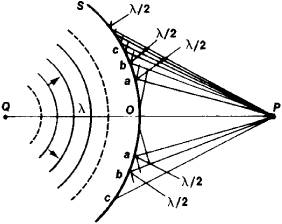Fresnel Zones

Fresnel zones

[frā′nel ‚zōnz]
(electromagnetism)
Circular portions of a wavefront transverse to a line between an emitter and a point where the disturbance is being observed; the n th zone includes all paths whose lengths are between n -1 and n half-wavelengths longer than the line-of-sight path. Also known as half-period zones.

Fresnel Zones

regions into which the surface of a light or sound wave may be divided to calculate the results of the diffraction of light or sound. The method was first used by A. Fresnel in 1815-19. The essence of the method is as follows: Let a spherical wave propagate from a luminous point Q (see Figure 1). The characteristics of the wave process induced by it at point P are to be determined. Let us divide the surface of the wave S into annular zones; for this pur-pose, spheres with radii PO, Pa = PO + λ/2; Pb = Pa + λ/2, and PC = Pb + λ/2 are constructed from point P (O is the point of intersection of the wave surface and the line PQ; λ is the length of the light wave). The annular regions of the wave surface that are “cut out” of the wave by these spheres are called Fresnel zones. The wave process at point P may be regarded as the result of the addition of the oscillations induced at this point by each individual Fresnel zone. The amplitude of these oscillations slowly decreases as the number of the zone (counted from point O) increases, and the phases of the oscillations induced at P by contiguous zones are opposite. Therefore, waves that arrive at P from two contiguous zones damp one another, whereas the action of zones separated by one zone is added. If the wave propagates without encountering obstacles., then, as shown by calculation, its action (the sum of the effects of all Fresnel zones) is equivalent to the action of half of the first zone. If the parts of the wave that correspond, for example, to N odd Fresnel zones are selected by means of a screen with transparent concentric regions, then the action of all selected zones will be added and the amplitude of the oscillations Uodd at point P will increase by 2N times and the intensity of the light by 4N2 times, and the illumination at the points surrounding P will decrease. The same effect occurs when only even zones are selected, but the phase of the composite wave Ueven will have the opposite sign.Figure 1

Such zone screens (so-called Fresnel lenses) are used not only in optics but also in acoustics and radio engineering—in the region of sufficiently short wavelengths, when the size of the lenses is not too great (centimeter radio waves and ultrasonic waves).

The method of Fresnel zones makes possible the rapid and graphic compilation of a qualitative idea, and sometimes a quite accurate quantitative idea, of the result of wave diffraction under various complex conditions of propagation. Therefore it is used not only in optics but also in the study of the propagation of radio and sound waves to determine the effective path of the “ray” as it moves from transmitter to receiver, to determine whether diffraction phenomena will play a role under specific conditions, to provide guidance in problems of the directivity of radiation, and to focus waves.

References in periodicals archive ?
In this work, we present the evaluation of the limits of validity of the numerical propagation methods through the reproduction of the results predicted in numerical experiments of diffraction by the fundamentals concepts of optics Babinet's principle and the number of Fresnel zones.
In this paper, in the section 2 a review of Babinet's principle and the number of Fresnel zones is done, after the propagation methods angular spectrum and Fresnel-Fraunhofer transform are explained and the utilized numerical experiment is described.
First, Babinet's principle and the number of Fresnel zones is exposed.
The number of Fresnel zones is a postulated by the French physicist Augustin-Jean Fresnel.
As metric we have used the predicted phase by Babinet's theorem for a geometry that subtend an even number of Fresnel zones.
However, the sieve is dotted with millions of holes precisely placed on silicon in a circular pattern that takes the place of conventional Fresnel zones.
The optic is a variant of something called a Fresnel zone plate.
As is well known, the use of reflectors or lenses based on diffractive Fresnel zones introduces a loss of gain and bandwidth when compared with parabolic reflectors.
When the family of Fresnel zones is based on concentric circumferences and independently of the illuminated area, the reflecting signal is perpendicular to the Fresnel surface (it is not necessary to illuminate complete zones to focalise the signal).
In this case, the family of Fresnel zones is based on ellipses , but as can be seen in the case of the Fresnel reflector which is shown in Figure 2, with the naked eye it is difficult to appreciate that the ellipses are indeed ellipses or circumferences.
One of them for example, is the active beam scanning capability which is described in Reference , in which it was demonstrated that a family of Fresnel zones based on concentric circumferences can focus signals from any angle of incidence.
The reflector has been designed with 6 Fresnel zones on two levels and it is etched on a square aluminium plate.

Site: Follow: Share:
Open / Close